Probability: Single Events Video for Kids - Grades 6-8
1%
It was processed successfully!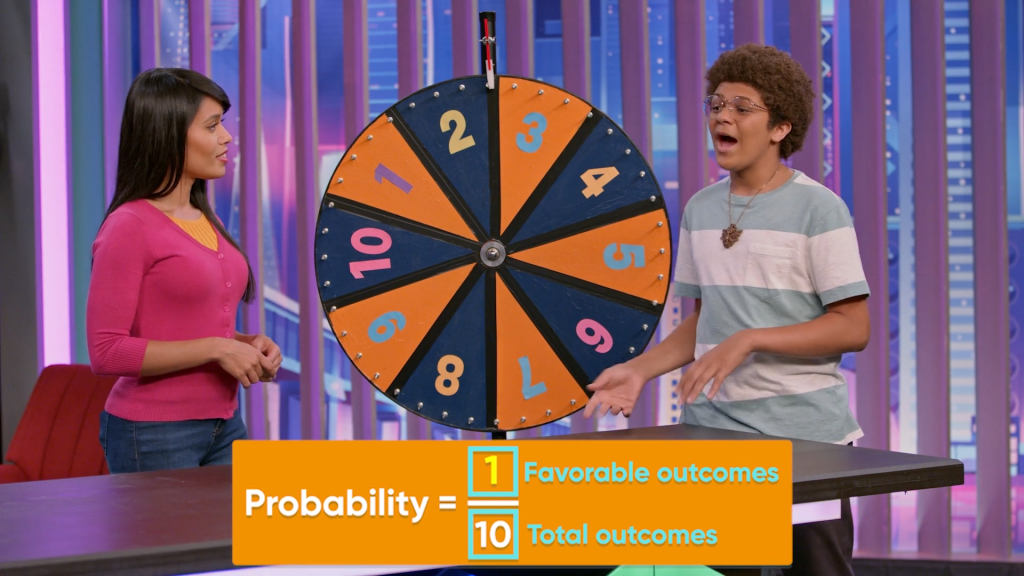WHAT IS PROBABILITY OF SINGLE EVENTS?

Probability tells us how likely it is that something will happen. We can write probability as a percent, a decimal, or a fraction. The lesser the probability, the less likely the event is to occur.

To better understand probability of single events…

WHAT IS PROBABILITY OF SINGLE EVENTS?. Probability tells us how likely it is that something will happen. We can write probability as a percent, a decimal, or a fraction. The lesser the probability, the less likely the event is to occur. To better understand probability of single events…

## LET’S BREAK IT DOWN!

### Find the probability of a coin toss.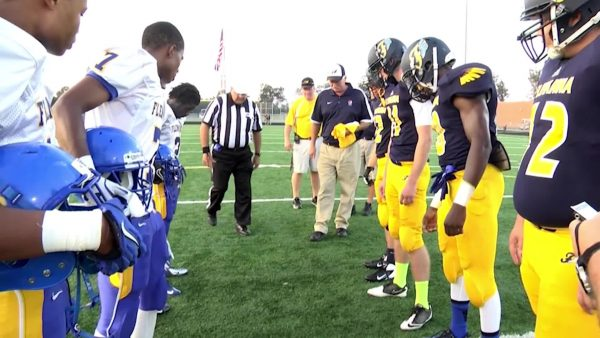A football match starts with a coin toss to decide which team starts with the ball. The coin has two sides, heads and tails. What is the likelihood, or the probability, that the coin turns up heads? The coin has two sides, so there are two possible outcomes of the coin toss. There is only one way for the coin to turn up heads. This means that the probability is 1 out of 2, [ggfrac]1/2[/ggfrac], 0.5, or 50%. The probability of tails is the same because there is only one way to come up with tails, out of two possible outcomes. Because the two events, “heads” and “tails,” have the same probability, they are equally likely. Try this yourself: A number cube has 6 sides, labeled 1 to 6. What is the probability of rolling a 1?

Find the probability of a coin toss. A football match starts with a coin toss to decide which team starts with the ball. The coin has two sides, heads and tails. What is the likelihood, or the probability, that the coin turns up heads? The coin has two sides, so there are two possible outcomes of the coin toss. There is only one way for the coin to turn up heads. This means that the probability is 1 out of 2, [ggfrac]1/2[/ggfrac], 0.5, or 50%. The probability of tails is the same because there is only one way to come up with tails, out of two possible outcomes. Because the two events, “heads” and “tails,” have the same probability, they are equally likely. Try this yourself: A number cube has 6 sides, labeled 1 to 6. What is the probability of rolling a 1?

### Probability tells you the likelihood of events.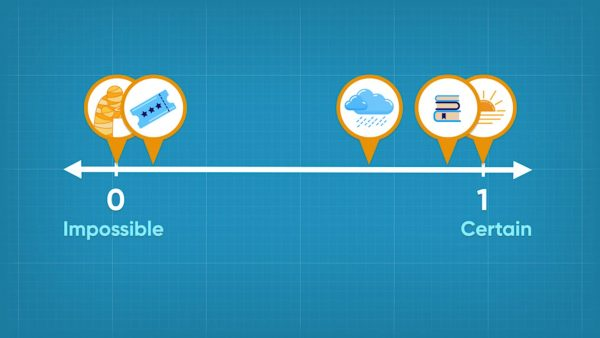Probabilities range in size from 0 to 1. You can describe how likely events are by their probability. If an event is impossible, it has a probability of 0. If an event is certain, it has a probability of 1. If the event is as likely to happen as it is to not happen, its probability is 0.5. If an event is not likely to happen, but still could happen, like winning the lottery, the probability is close to 0. If an event is very likely to happen but might not happen, like having homework after school today, its probability is close to 1. Try this yourself: On a number line from 0 to 1, with 0 being impossible and 1 being certain, how likely is it that it will rain today? Check the weather forecast: what probability do they give for rain today?

Probability tells you the likelihood of events. Probabilities range in size from 0 to 1. You can describe how likely events are by their probability. If an event is impossible, it has a probability of 0. If an event is certain, it has a probability of 1. If the event is as likely to happen as it is to not happen, its probability is 0.5. If an event is not likely to happen, but still could happen, like winning the lottery, the probability is close to 0. If an event is very likely to happen but might not happen, like having homework after school today, its probability is close to 1. Try this yourself: On a number line from 0 to 1, with 0 being impossible and 1 being certain, how likely is it that it will rain today? Check the weather forecast: what probability do they give for rain today?

### Find the probability of outcomes on a wheel.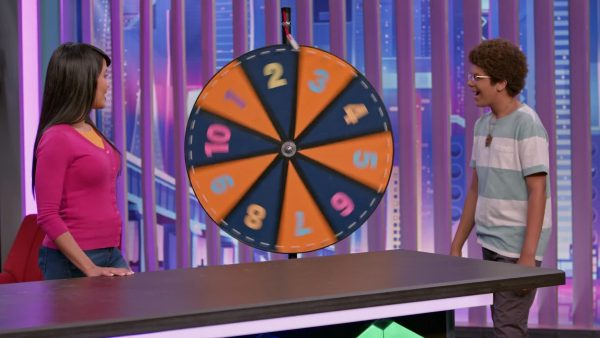A game wheel has 10 equal sections, labeled with numbers 1 to 10. This means there are 10 possible outcomes. There is only one way to spin a 2. This means that the probability of spinning a 2 is 1 out of 10. You can also write this as [ggfrac]1/10[/ggfrac], 0.1, or 10%. What is the probability a spin lands on a number (it doesn't matter which one)? There are 10 ways to spin any number and 10 possible outcomes. The probability of spinning any number is 10 out of 10, [ggfrac]10/10[/ggfrac], 1, or 100%. This means the event “spin any number” is certain. It also makes sense because you land on a number 100% of the time. Try this yourself: If the wheel has 25 equal sections, labeled with numbers 1 to 25, what is the probability of spinning a 7? What is the probability of spinning a number 15 or greater?

Find the probability of outcomes on a wheel. A game wheel has 10 equal sections, labeled with numbers 1 to 10. This means there are 10 possible outcomes. There is only one way to spin a 2. This means that the probability of spinning a 2 is 1 out of 10. You can also write this as [ggfrac]1/10[/ggfrac], 0.1, or 10%. What is the probability a spin lands on a number (it doesn't matter which one)? There are 10 ways to spin any number and 10 possible outcomes. The probability of spinning any number is 10 out of 10, [ggfrac]10/10[/ggfrac], 1, or 100%. This means the event “spin any number” is certain. It also makes sense because you land on a number 100% of the time. Try this yourself: If the wheel has 25 equal sections, labeled with numbers 1 to 25, what is the probability of spinning a 7? What is the probability of spinning a number 15 or greater?

### The sum of all possible probabilities is 1 or 100%.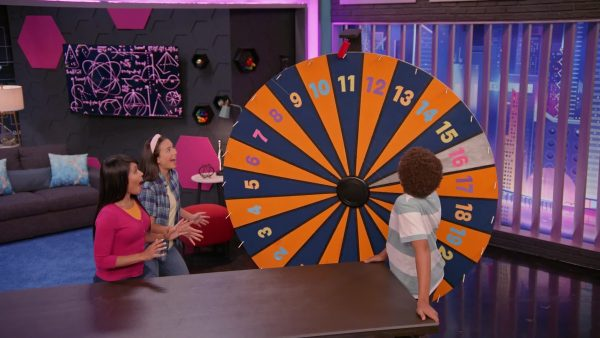On the original game wheel, the probability of spinning a specific number is [ggfrac]1/10[/ggfrac]. The probability of spinning any number at all is 1. The sum of the probabilities of spinning each number is 1. So the probability of spinning any number on the wheel is the same as [ggfrac]1/10[/ggfrac] + [ggfrac]1/10[/ggfrac] +[ggfrac]1/10[/ggfrac] + [ggfrac]1/10[/ggfrac] + [ggfrac]1/10[/ggfrac] + [ggfrac]1/10[/ggfrac] + [ggfrac]1/10[/ggfrac] + [ggfrac]1/10[/ggfrac] + [ggfrac]1/10[/ggfrac] + [ggfrac]1/10[/ggfrac] = [ggfrac]10/10[/ggfrac] = 1. Try this yourself: If the wheel has 25 equal sections, labeled with numbers 1 to 25, what is the probability of spinning any of the 25 numbers on the wheel?

The sum of all possible probabilities is 1 or 100%. On the original game wheel, the probability of spinning a specific number is [ggfrac]1/10[/ggfrac]. The probability of spinning any number at all is 1. The sum of the probabilities of spinning each number is 1. So the probability of spinning any number on the wheel is the same as [ggfrac]1/10[/ggfrac] + [ggfrac]1/10[/ggfrac] +[ggfrac]1/10[/ggfrac] + [ggfrac]1/10[/ggfrac] + [ggfrac]1/10[/ggfrac] + [ggfrac]1/10[/ggfrac] + [ggfrac]1/10[/ggfrac] + [ggfrac]1/10[/ggfrac] + [ggfrac]1/10[/ggfrac] + [ggfrac]1/10[/ggfrac] = [ggfrac]10/10[/ggfrac] = 1. Try this yourself: If the wheel has 25 equal sections, labeled with numbers 1 to 25, what is the probability of spinning any of the 25 numbers on the wheel?

### Compare experimental and theoretical probabilities.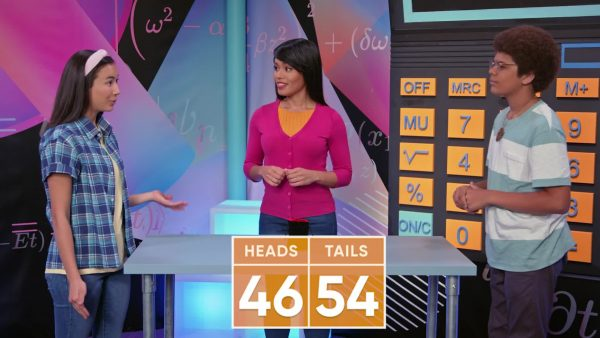You flip a coin 10 times. You expect to get 5 heads and 5 tails, because the probability of the coin landing on each side is [ggfrac]1/2[/ggfrac], or 50%. The result of your flips is 6 heads and 4 tails. Why didn’t you get 5 heads and 5 tails? The probability, 50%, is the theoretical probability. The results of your experiment are a probability of 60% heads and 40% tails. This is the experimental probability. It is the result of an experiment. As you do more and more flips of the coin, the experimental probability will get closer to the theoretical probability. This effect is called the Law of Large Numbers. Try this yourself: If you flip a bottle 100 times, and in 20 of the trials the bottle lands on its base, what is the experimental probability of the bottle landing on its base?

Compare experimental and theoretical probabilities. You flip a coin 10 times. You expect to get 5 heads and 5 tails, because the probability of the coin landing on each side is [ggfrac]1/2[/ggfrac], or 50%. The result of your flips is 6 heads and 4 tails. Why didn’t you get 5 heads and 5 tails? The probability, 50%, is the theoretical probability. The results of your experiment are a probability of 60% heads and 40% tails. This is the experimental probability. It is the result of an experiment. As you do more and more flips of the coin, the experimental probability will get closer to the theoretical probability. This effect is called the Law of Large Numbers. Try this yourself: If you flip a bottle 100 times, and in 20 of the trials the bottle lands on its base, what is the experimental probability of the bottle landing on its base?

## PROBABILITY: SINGLE EVENTS VOCABULARY

Probability
The extent to which an event is likely to occur. Probability is expressed using numbers between 0 and 1.
Impossible
An event that cannot happen is impossible. Events that are impossible have a probability of 0.
Likely
An event is likely if we are pretty sure it will happen, but it still may not happen. Likely events have probabilities close to 1.
Unlikely
An event is unlikely if we are pretty sure it won’t happen, but it still may happen. Unlikely events have probabilities close to 0.
Certain
An event that will happen for sure is certain. Events that are certain have a probability of 1.
Equally likely
If two events have the same probability, then the events are equally likely. If two outcomes have the same probability, then the outcomes are equally likely.
A set of possible outcomes that result from a particular experiment or situation. For example, when rolling a number cube to move a playing piece, the event “roll 4 or higher” includes the set of outcomes {4, 5, 6}.
The result of an experiment. For example, when determining the experimental probability of getting heads on a coin flip, each coin flip is a trial.
Any potential result in an experiment. For example, when flipping a coin there are two outcomes: {heads, tails}; when rolling a number cube there are 6 outcomes: {1, 2, 3, 4, 5, 6}.
The number of times an event happens during an experiment is the frequency of the event.
The product of the theoretical probability and the number of trials in the experiment gives the expectation, or expected frequency, for an event in that experiment.
A probability determined by experimental trials.
A probability that is calculated. For events with equally likely outcomes, the theoretical probability is the number of ways the event can happen divided by the total number of outcomes in the sample space.
The set of all possible outcomes in an experiment.
As the number of trials in an experiment increases, the experimental probability gets closer to the theoretical probability. So, if you want to determine the probability of an event using an experiment, you need a lot of trials to get an accurate value of the experimental probability.

## PROBABILITY: SINGLE EVENTS DISCUSSION QUESTIONS

### What is a probability?

Probability is the extent to which something is likely to occur. Probabilities have values between 0 and 1, describing likelihoods from impossible (0) to certain (1).

### What is a probability model?

A probability model describes how probability is distributed amongst all possible outcomes in a sample space. In a uniform probability model, all outcomes are equally likely. In a non-uniform probability model, some outcomes are more likely than others.

### What is theoretical probability?

A theoretical probability is one you calculate based on the number of outcomes possible and the number of outcomes in the event.

### What is experimental probability?

An experimental probability is one you calculate by conducting an experiment and determining the frequency of an event in a fixed number of trials.

### Which is more likely, a 0.2 chance it will rain, or a 0.6 chance it will snow?

Likelihood increases with larger numbers. Since 0.6 is larger than 0.2, it is more likely to snow than to rain.
X

## Success

We’ve sent you an email with instructions how to reset your password.
Ok